Controlling The Real World With Computers
::. Control And Embedded Systems .::

 Home Order Let me know what you think
Previous: Address Lines And Ports Next: Hardware

In general terms, a circuit can be described as any group of electrical or electronic devices connected together by conductors. Conductors are most often metallic, and wires were the conductor of choice in the past. Old radios and other electronic equipment were often a rat's nest of wires. Today, it's more common to find metallic pathways, often called traces, on a board constructed of a mixture of fiberglass and epoxy. The terms board and card are interchangeable.

A schematic in electronics is a drawing representing a circuit. It uses symbols to represent real-world objects. The most basic symbol is a simple conductor, shown simply as a line. If wires connect in a diagram, they are shown with a dot at the intersection: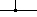Conductors that do not connect are shown without a dot, or with a bridge formed by one wire over the other:Among the connections are power and ground, the high and low system voltages respectfully. The 5 volt system power in the schematic is shown simply as 5V. There is also a +12V supply and a -12V supply. Ground, or 0 volts, has its own symbol:A switch is a device that is capable of allowing the user to break the circuit as if the wire had been broken. Its symbol reflects this characteristic: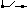The three switches in the diagram are grouped in a Dual In-line Package (DIP).

A resistor is a device that resists the flow of charge. Its symbol reflects this characteristic by making the line jagged: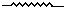Just in case you have seen "flow of current" elsewhere rather than "flow of charge", see "Science Myths" in K-6 Textbooks and Popular culture and the definition of current below.

The unit of resistance is the ohm, pronounced om with a long o. The K in the schematics stands for kilohm or thousands of ohms. 10K means the same as 10,000. Meg and sometimes M mean megohm or million ohms. 4.7Meg or 4.7M is the same as 4,700,000.

You will see two variations on resistors in the schematic. One is the resistor array or network. It is a Single In-line Package (SIP) containing several resistors connected together. They can be found in many configurations. The one used here simply connects one end of the resistors to each other and brings them out to a common connection. The other end of each resistor is left free. Another variation is the variable resistor. It has a third contact that can move along the resistor element to permit the values at that point to be variable. The moveable part is called the wiper and is shown as an arrow.

There is a relationship between voltage, current and resistance that is expressed by Ohm's Law, which says that Voltage is equal to Current times Resistance, or:

V = I * R

V is voltage (often referred to as Electromotive Force where E rather than V is used), I is current and R is resistance. Current is expressed in Amperes, or amps for short. Very little current is used in typical electronic circuits, so milliamps, which means 1/1000 amp, is used. One milliamp = .001 amp. It's abbreviated ma, or sometimes MA.

To paraphrase a definition of charge from whatis.com :

"The coulomb (symbolized C) is the standard unit of electric charge in the International System of Units (SI). It is a dimensionless quantity. A quantity of 1 C is equal to approximately 6.24 x 1018, or 6.24 quintillion."

"In terms of SI base units, the coulomb is the equivalent of one ampere-second. Conversely, an electric current of 1 amp represents 1 C of unit electric charge carriers flowing past a specific point in 1 second. The unit electric charge is the amount of charge contained in a single electron. Thus, 6.24 x 1018 electrons have 1 C of charge. This is also true of 6.24 x 1018 positrons or 6.24 x 1018 protons, although these two types of particles carry charge of opposite polarity to that of the electron."

Since we deal mostly with electrons in electronics, 1 amp represents the effect of 6,240,000,000,000,000,000 electrons flowing past a point per second. Thus, since current is already defined as something flowing, to say "current flow" would be to say "..... flowing flow" which is incorrect because it is redundant.

Now let's say we have a 10K resistor and 2 milliamps of current. The voltage across the resistor will be:

V = 10,000 * .002 = 20 volts

We can use the above equation to generate an equation for each of the three variables. It requires remembering just two things:
1. It's ok to do something to one side of an equation as long as the same thing is done to the other side. The two sides will remain equal.
2. Anything divided by itself is equal to 1.

V = I * R
Now divide both sides by R. Since R/R = 1, the right side now becomes I * 1 which is simply I, giving us V/R = I. If we switch sides and put the I on the left we end up with:
I = V/R

V = I * R
Now divide both sides by I. Since I/I = 1, the right side now becomes R * 1 which is simply R, giving us V/I = R. If we switch sides and put the R on the left we end up with:
R = V/I

Thus, all three equations are:
V = I * R
I = V/R
R = V/I

One way to remember the three equations is to say, "The Vulture looks down and sees the Iguana and the Rabbit side by side (V = I * R), the Iguana sees the Vulture over the Rabbit (I = V/R) and the Rabbit sees the Vulture over the Iguana (R = V/I)."A very common circuit is a voltage divider. It looks like the following: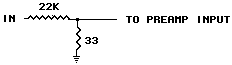Two resistors connected end-to-end are said to be connected in series. The total resistance is simply the sum of the two. In this case, it would be 22000 + 33 = 22033 ohms. If 1 volt is applied to the open end of the 22K resistor, the current through the whole circuit would be
I = V/R = 1/22033 or .00004538646576 amps, or about .05 milliamps.

The voltage across the 33 ohm resistor is then
V = I * R = .00004538646576 * 33 = .00149775337 volts, or about 1.5 millivolts (1/1000 volt).

Resistors are also often connected in parallel , such as below:The value of the above parallel network is:
R = 1/(1/R1 + 1/R2 + 1/R3)
The equation is good for any number of resistors.

Capacitors are devices which have metal plates separated by an insulator. They are used to temporarily store an electrical charge. Their symbol reflects their construction: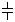The unit of capacitance is the Farad, but it's so large that the microfarad is used in practice. Microfarad means millionths of a Farad. It's often abbreviated mf, MF or some variation, although the correct abbreviation is µF. A value without a designator is assumed to be in microfarads. For example, in the schematic you will see several capacitors simply designated .1. They are actually .1µF capacitors.

Some capacitors must have their leads connected to the positive or negative side of a circuit. They are polarized capacitors. When that is the case, one side will be shown with a + sign where the positive side must be, or a - sign where the negative side must be, or both.

It's also very common to see picofarads abbreviated pf in some schematics. A picofarad is 10-12 Farad, and is sometimes called a micromicrofarad.

A diode permits the flow of charge in only one direction. Its symbol reflects this characteristic, but with a slight problem:

Anode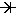Cathode

The slight problem comes from the fact that flow of charge, at least in a wire, is from where there are a greater number of electrons to where there are fewer. Electrons are negatively charged. Thus, electrical flow of charge is from negative to positive in a wire. The problem with the symbol is that the cathode, not the anode, is the negative side. Electrical flow of charge is from the cathode to the anode, against the direction of the arrow.

Integrated Circuits contain many individual components. They, in turn, usually form several functional blocks. For example, the following is a pinout for the 74LS08 Quad 2 Input AND gate, along with its truth table. VCC is the 5 volt supply, and GND is ground. Sometimes ground is shown as VSS. The gate inputs are the As and Bs, and the outputs are the Ys. Thus, the inputs to gate 1 are 1A and 1B, and the output is 1Y. You will see variations on these conventions, but they hold true in many cases.An Operational Amplifier also contains many individual components, but is not a digital circuit. It looks a little like a buffer, but has 2 inputs:You can find a more detailed treatment of operational amplifiers at Professor Douglas M. Gingrich's site at The University of Alberta. For a simplified coverage of the subject, look at the circuit below.An Op-Amp has many important characteristics. One of them is that the above circuit, called an inverting amplifier, attempts to prevent any current through the inverting input. In this circuit, R1 connects to the inverting input. R2 also connects to the inverting input, with its other end connected to the output. R2 is called the feedback resistor. Let's attempt to drive a current through the inverting input by placing 1V on the unconnected end of R1 and assume that the right end has 0 volts on it. The current will be
I = V/R = 1/1K = 1ma

The output will try to counter this by driving a current of the opposite polarity through the feedback resistor into the inverting input. The required voltage to do that will be
V = -(I * R) = -(1ma * 10K) = -10V.

Thus, we get a voltage to current conversion, a current to voltage conversion, a polarity inversion and, most importantly, amplification. Amplification or gain is commonly labeled G. In the case of the inverting amplifier,
G = -(Feedback Resistor / Input Resistor)
In this case, it's G = -(R2/R1)

Since the feedback cancels out the input, there is no voltage at the inverting input. It is said to be at virtual ground .

Now look at the circuit below from the schematic you will see in the hardware section.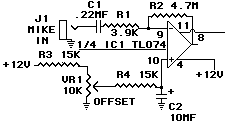The gain is a little over -1000 in order to provide enough amplification for the low output of a microphone. The signal is not only amplified but inverted because we are going into the inverting input. The inversion however, is not quite the same as it is in a digital device. Here, we are talking about an audio analog signal that, once transformed into an electrical signal by the microphone, moves much more smoothly and continuously in the negative and positive voltage directions. Inversion here means that when the input moves in the positive direction, the output moves in the negative direction. When the input goes toward negative, the output goes toward positive. C1 prevents DC voltages from even getting into the circuit. This blocking action will be discussed in a future section.

The non-inverting side is designated by the +. It is there that a positive offset voltage is applied. If R1 were not connected to C1 but rather to ground, the non-inverting side would exhibit a gain of (R2/R1)+1 for the bias voltage. With C1 however, there is no DC gain for the non-inverting side, and AC is shorted to ground by C2. The result is a gain of 1 on the non-inverting side for DC voltages. The purpose of the bias circuit will be covered in the next section.

The following is a self-test over this section. It would be a very good idea to make sure you know the answers to all of the questions since the sections that follow will build on this one.

1) _____ is a drawing that represents a circuit.

A) Switch
B) Schematic
C) Ground
D) Diagram

2) A _____ is a device that allows the user to break the circuit.

A) Scissors
B) Schematic
C) Resistor
D) Switch

3) A _____ is a device that resists the flow of charge.

A) Resistor
B) Buffer
C) Diode

4) The unit of resistance is the __1__ . The relationship between voltage, current, and resistance is expressed by __2__ .

A) Buffer, Amplifier
B) Capacitors, Diode
C) Ohm, Ohm's Law
D) Circuits, Switch

5) The __1__ is the unit of current. If there is very little current, it is expressed as __2__, which means 1/1000th.

A) Amperes (or Amps), Milliamps (or Ma or ma)
B) Volts, Milliavolts
D) Amplifier, Circuits

6) _____ are devices which have metal plates separated by an insulator. They temporarily store an electrical charge.

A) In Series
B) Cathode
C) Capacitors

7) What permits the flow of charge in only one direction?

A) Anode
B) Diode
C) Cathode
D) Schematic

8) _____ contain many individual components and usually form several functional blocks.

A) Schematics
B) Diodes
C) Amplifiers
D) Integrated Circuits

9) The _____ also contains many components, but is not a digital device.

A) Inverting Amplifier
B) Operational Amplifier
C) Volt
D) Electron

10) Thisis __________________________________________

11) Thisis __________________________________________

12) Thisis __________________________________________

13) Thisis __________________________________________

14) Thisis __________________________________________

15) Thisis __________________________________________

16) Thisis __________________________________________

17) Ohm's Law: __________________________________________

18) I = 4, R = 10 so V = ________________ ________________

19) V = 12, R = 6 so I = ________________ ________________

20) I = 75, V = 150K Volts so R = ________________ ________________

Previous: Address Lines And Ports ---- Next: Hardware